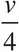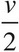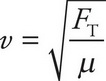# AP Physics 1 Question 410: Answer and Explanation

### Test Information

Question: 410

8. The speed of a transverse wave on a stretched string is v. If the string is replaced with one half of the linear density and the tension in the string is doubled, what is the new speed of the transverse wave?

• A.• B.• C. v
• D. 2v

The speed of a transverse wave in a stretched string is given by the equation, where FT is the tension in the string and μ is the linear mass density. Doubling FT and decreasing μ by a factor of 2 will result in an increase of v by a factor of 2.